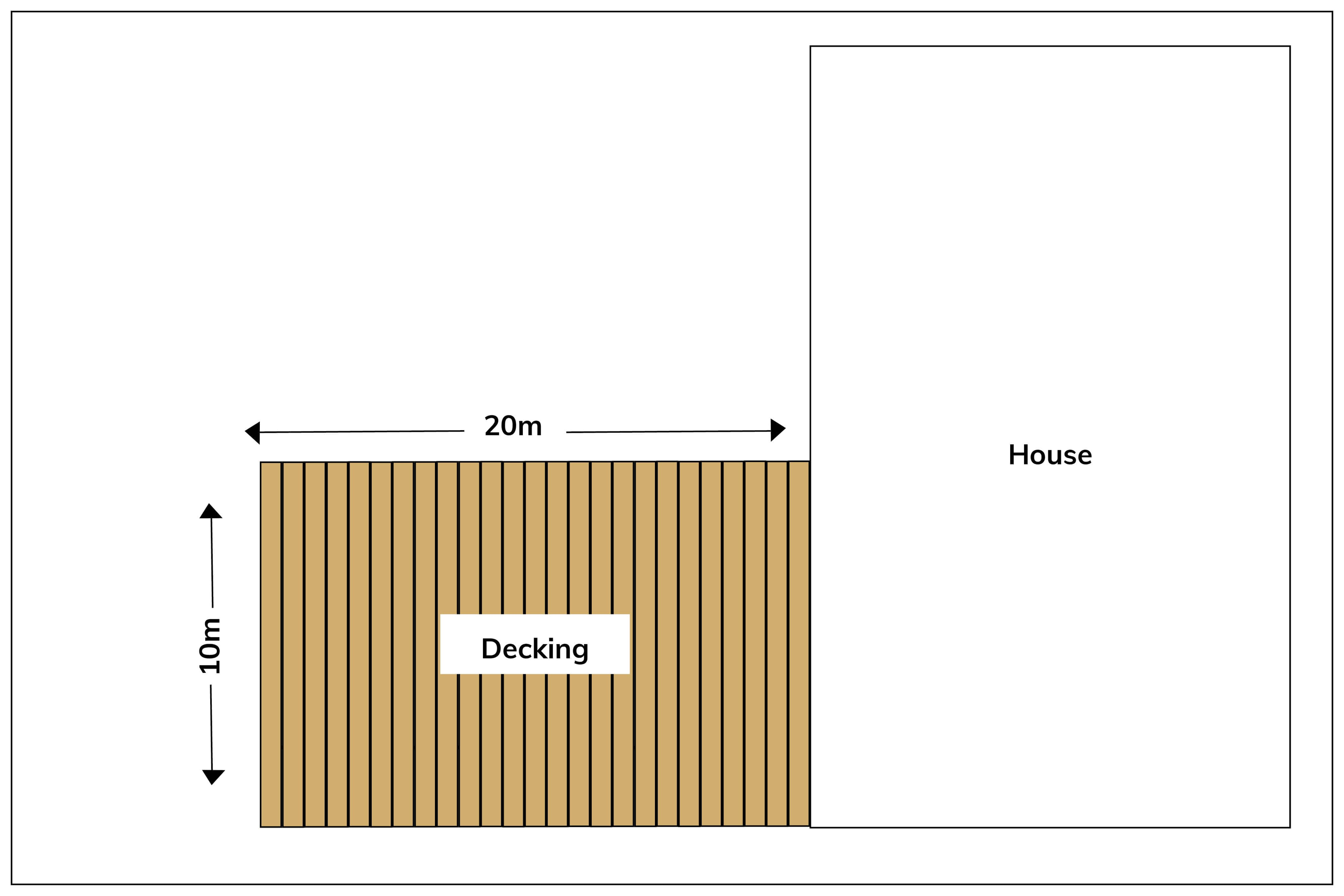# Decking Calculator

Once you’ve decided on the size, design and materials for your garden decking, you’ll need to work out how many deck boards to order. The simplest way to estimate this, is by using the following calculation:

STEP 1: Measure the length and width of the area you wish to deck and multiply these together. This gives you a square metre area.

For example, 20 x 10 = 200m²

STEP 2: Take this value and divide by the width of the board as a decimal to calculate how many linear metres you require.

For example, Gripsure Home has a width of 125mm, therefore your calculation would be 200 ÷ 0.125 = 1600 linear metres

STEP 3: Divide your linear metre total by the length of board you wish to order to work out the total number of boards needed.

For example, 1600 ÷ 3.6 = 445 (figure rounded to the nearest whole)

STEP 4: We always suggest factoring in some cutting and wastage. This will vary depending on the size and shape of the deck. As a general rule, we recommend allowing for an additional 5-10%.

For example, 445 boards + 10% = 490 boards to order in total (number rounded to the nearest whole)Top Tip

This calculation is for horizontal decking designs only and includes the expansion gaps required (approx. 5-8mm). For any additional help calculating your decking area please call us on 01726 844616.